## A relay microchip in a telecommunications satellite has a life expectancy that follows a normal distribution with a mean of 90 months and a

Question

A relay microchip in a telecommunications satellite has a life expectancy that follows a normal distribution with a mean of 90 months and a standard deviation of 3.5 months. When this computer-relay microchip malfunctions, the entire satellite is useless. A large London insurance company is going to insure the satellite for 50 million dollars. Assume that the only part of the satellite in question is the microchip. All other components will work indefinitely.
A. For how many months should the satellite be insured to be 94% confident that it will last beyond the insurance date?
B. If the satellite is insured for 84 months, what is the probability that it will malfunction before the insurance coverage ends?
C. If the satellite is insured for 84 months, what is the expected loss to the insurance company?
D. If the insurance company charges $3 million for 84 months of insurance, how much profit does the company expect to make? in progress 0 6 months 2021-08-19T19:14:10+00:00 1 Answers 0 views 0 ## Answers ( ) 1. Answer: a) The insurance should be of 84.6 months, that is, approximately 85 months. b) 0.0436 = 4.36% probability that it will malfunction before the insurance coverage ends. c) The expected loss to the insurance company is of 2.18 million. d) The company should expect to make a profit of$689,200.

Step-by-step explanation:

Normal Probability Distribution:

Problems of normal distributions can be solved using the z-score formula.

In a set with mean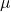and standard deviation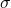, the zscore of a measure X is given by: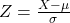The Z-score measures how many standard deviations the measure is from the mean. After finding the Z-score, we look at the z-score table and find the p-value associated with this z-score. This p-value is the probability that the value of the measure is smaller than X, that is, the percentile of X. Subtracting 1 by the pvalue, we get the probability that the value of the measure is greater than X.

A relay microchip in a telecommunications satellite has a life expectancy that follows a normal distribution with a mean of 90 months and a standard deviation of 3.5 months.

This means that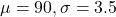A. For how many months should the satellite be insured to be 94% confident that it will last beyond the insurance date?

The 100 – 94 = 6th percentile, which is X when Z has a pvalue of 0.06. So X when Z = -1.555.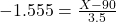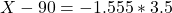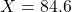The insurance should be of 84.6 months, that is, approximately 85 months.

B. If the satellite is insured for 84 months, what is the probability that it will malfunction before the insurance coverage ends?

This is the pvalue of Z when X = 84. So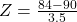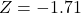has a pvalue of 0.0436

0.0436 = 4.36% probability that it will malfunction before the insurance coverage ends.

C. If the satellite is insured for 84 months, what is the expected loss to the insurance company?

0.0436 probability of losing 50 million. So

0.0436*50 = 2.18

The expected loss to the insurance company is of 2.18 million.

D. If the insurance company charges $3 million for 84 months of insurance, how much profit does the company expect to make? 1 – 0.0436 = 0.9564 probability of making 3 million. 0.0436 probability of losing 50 million So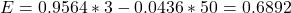The company should expect to make a profit of 0.6892 million, that is,$689,200.